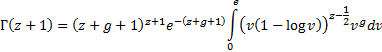/

### The Lanczos Approximation

The Lanczos approximation is a method for computing the gamma function numerically, originally derived by Cornelius Lanczos in 1964 and involving the following formula: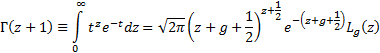Here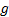is an arbitrary constant subject to the restriction that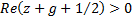and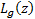and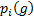are as follows, where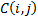is the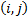’th element of the Chebyshev polynomial coefficient matrix: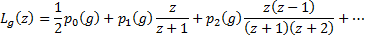The series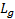converges. By choosing an appropriate, typically a small positive number, only a few terms are needed to calculate the gamma function to a high degree of accuracy. The series approximation can then be recast into the following form, with the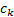calculated in advance: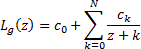According to Wikipedia (2015), Lanczos derived the formula by deriving the following integral representation for the gamma function and then deriving a series expansion for the integral within this representation: